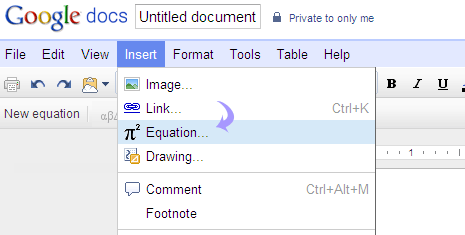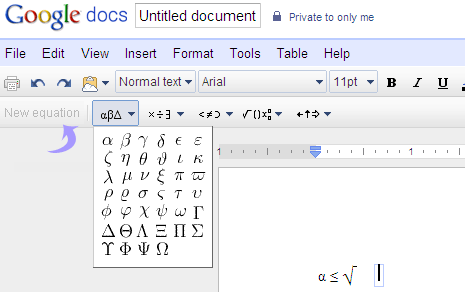# Insert Math symbols & equations in Google Docs

Do you want to add and display complex Mathematical equations in Google Docs files? You can easily do this using “Equation” feature. It allows you to insert any of commonly used mathematical signs and symbols to your Google documents in few simple clicks.

## Mathematical symbols, equations in Google Docs

2. Click ‘Create New’ to start new document or open any existing document file.3. Then goto Insert > Equation to view equation toolbar.

4. Use equation toolbar to add either of: Greek letters, General operators, Comparison and inclusion operators, Operators with variables and Arrows.5. If equation toolbar is not visible goto View > Show equation toolbar to show the equation toolbar.

Besides adding new math equations, you can edit previous equations anytime by clicking that part of the document.

SHARE